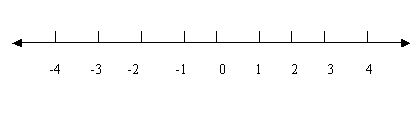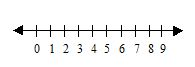Email us to get an instant 20% discount on highly effective K-12 Math & English kwizNET Programs!

#### Online Quiz (WorksheetABCD)

Questions Per Quiz = 2 4 6 8 10A numberline shows the numbers in order. Numbers to the right of zero are positive numbers. Numbers to the left are negative numbers. A numberline has arrows because the numbers keep going forever. You can use the numberline to practice the addition and subtraction.Method: Draw a number line with numbers. To find difference, we first go forward till we reach the bigger number and then backward till we reach the smaller number. To find sum, we first go till the first number and then move further from there to reach the next number. Directions: Answer the following questions. Also write at least ten examples with subtraction and addition using number line.
 Q 1: To find the difference of 9 - 5 on the number line, first go forward to 9 then go backward 5 numbers. We end up at _____.9647 Q 2: On a number line, what is five more of number Nine?16141312 Q 3: A number line shows numbers in order.TrueFalse Q 4: Negative numbers are the numbers to the left of zero on a number line.FalseTrue Q 5: On a number line, what is four more of number seven?7121110 Q 6: On a number line the numbers are in order. falseTrue Q 7: Positive numbers are numbers on the right of zero on the number line.TrueFalse Q 8: On a number line, what is four less than seven?5436 Question 9: This question is available to subscribers only! Question 10: This question is available to subscribers only!